# ODE Cash Karp 5th Order VI

## LabVIEW 2018 Help

Edition Date: March 2018
Part Number: 371361R-01
View Product InfoLabVIEW 2016 HelpLabVIEW 2017 HelpLabVIEW 2018 HelpLabVIEW 2019 HelpLabVIEW 2020 Help

Owning Palette: Ordinary Differential Equations VIs

Requires: Full Development System

Solves ordinary differential equations with initial conditions using the Cash Karp method.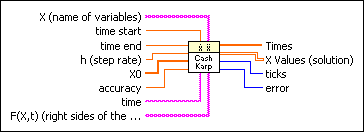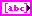X is an array of strings of variables.time start is the start point of the ODE. The default is 0.time end is the end point of the time interval under investigation. The default is 1.0.h is the step rate at the beginning of the algorithm. The Cash Karp algorithm works with an adaptive step rate. The default is 0.1.X0 is the vector of the start condition x, …, x[n0]. There is a one-to-one relation between the components of X0 and X.accuracy controls the accuracy of the solutions. The default is 0.0, which specifies the maximum deviation of the calculated solution from the actual solution.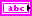time is the string denoting the time variable. The default variable is t.F(X,t) is a 1D array of strings representing the right sides of the differential equations. The formulas can contain any number of valid variables.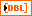Times is a 1D array representing the time steps. The ODE Cash Karp method yields arbitrarily chosen time steps between time start and time end.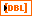X Values is a 2D array of the solution vector x, …, x[n]. The top index runs over the time steps, as specified in the Times array, and the bottom index runs over the elements of x, …, x[n].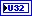ticks is the time in milliseconds for the whole calculation.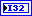error returns any error or warning from the VI. Errors are produced by using the wrong inputs X, X0, and F(X,t). You can wire error to the Error Cluster From Error Code VI to convert the error code or warning into an error cluster.

## ODE Cash Karp 5th Order Details

The Cash Karp method works with an adaptive step rate and is computationally more efficient than the Euler method and the Runge Kutta method. The Cash Karp method is an embedded Runge Kutta formula and is based on a fifth-order strategy (with six steps).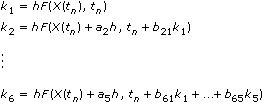and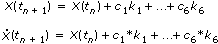with

tn + 1 = tn + h

The a2, …, a6; b21, …, b65; c1, …, c6; and c1*, …, c6* are fixed real numbers. This choice determines the quality of the method.

You can determine the actual step size hnew with the help of the accuracy value, the old step size h, the difference= |X(tn + 1) – X*(tn + 1)|, and the following equation.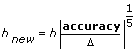Note  It might happen that the value of the last element in Times turns out to be greater than the value entered in time end. This is a property of the Cash Karp method. This method is very accurate, but you have no control of the step rate. In order to guarantee that the end point specified in time end is taken into consideration, the last step might turn out to be too long.

The following illustration shows the solution of the following system of ordinary differential equations in a 3D representation.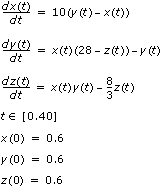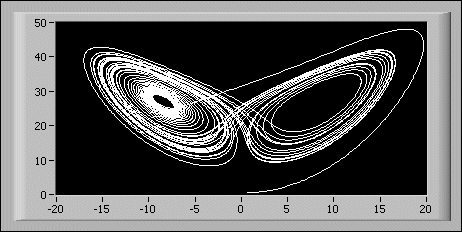The previous equations and boundary conditions are entered on the front panel as:

• time start: 0.00
• time end: 40.00
• X0: [0.6, 0.6, 0.6]
• F(X,t): [10*(y-x), x*(28-z) - y, x*y - (8/3)*z]
• X: [x,y,z]

## Example

Refer to the Planar Three Body Problem VI in the labview\examples\Mathematics\Differential Equations - ODE directory for an example of using the ODE Cash Karp 5th Order VI.